Courses
Courses for Kids
Free study material
Free LIVE classes
More

# V-I Characteristics## Introduction of V-I Characteristics

The voltage-current characteristics of an electrical component or device are referred to as V-I characteristics. The V-I graph provides information about resistance and breaks down an electronic component. It also specifies a component's operating region. By studying its characteristics, we can learn where and how to use a component in an electric circuit.

An electronic component's voltage-current characteristics describe its behaviour for various applied voltage values. Simply put, it is the graph obtained when current is measured through an electronic component while a voltage is applied across it. Because it is easier to control the applied voltage rather than the current. In V-I characteristics, the voltage is considered the independent variable.

## Linear VI Characteristics

Ohmic resistors display linear V-I properties. Ohm's law discusses the relationship between voltage and current, stating that voltage and current are proportional to each other, resulting in a straight line when a graph is drawn. The line's slope provides resistance. The greater the slope, the greater the resistance.

Hence,

$R=\dfrac {\Delta V}{\Delta I}~and~R=\tan q$

The V-I properties result in a straight line going through the origin. If the voltage polarity is switched in a resistor, the current will begin to flow in the other direction with equal magnitude if the magnitude of the voltage is not changed.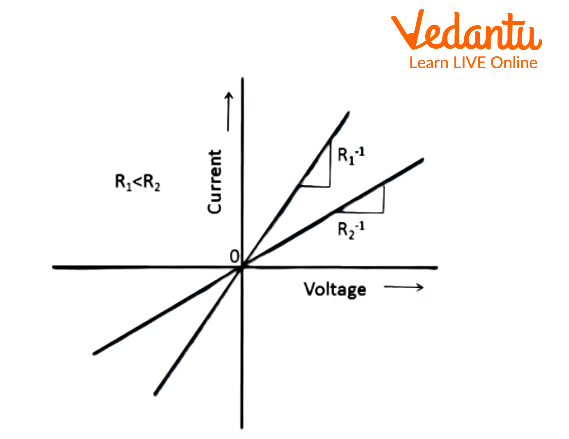Linear VI Characteristics

## Non-linear V-I Characteristics

If non-linear V-I characteristics exist, as they do, resistance must have a different property. If the V-I graph is not linear, the resistance is not constant and depends on either voltage or current. Diodes, SCRs (Silicon controlled resistors), transistors, and other non-ohmic resistance devices are examples. These are the most noticeable changes in non-linear graphs.

• Voltage and current are no longer proportional.

• Voltage determines the relationship between voltage and current when the voltage is reversed without changing the magnitude, the current reverses but the magnitude changes.

• Because the relationship between V and I is not unique, more than one voltage value can be obtained for a given value of current.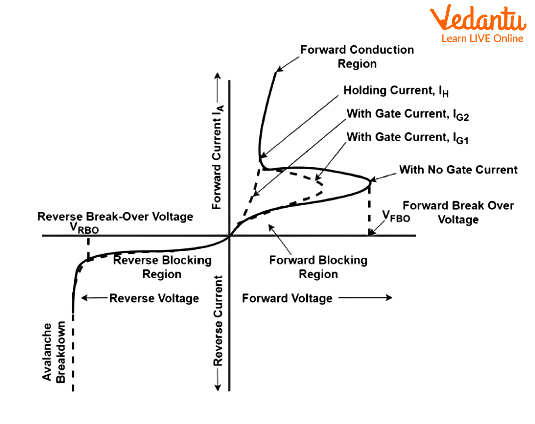Non-Linear VI Characteristics

## V-I Characteristics of SCR

The SCR (Silicon Controlled Rectifier) V-I Characteristics is a graphical representation of current through the SCR and voltage across the anode to cathode terminal. SCRs are three-terminal semiconductor switching devices that regulate and invert power flows. Based on its V-I characteristics, an SCR has three primary modes of operation: reverse blocking mode, forward blocking mode, and forward conduction mode. SCRs are also known as thyristors or thyroid transistors.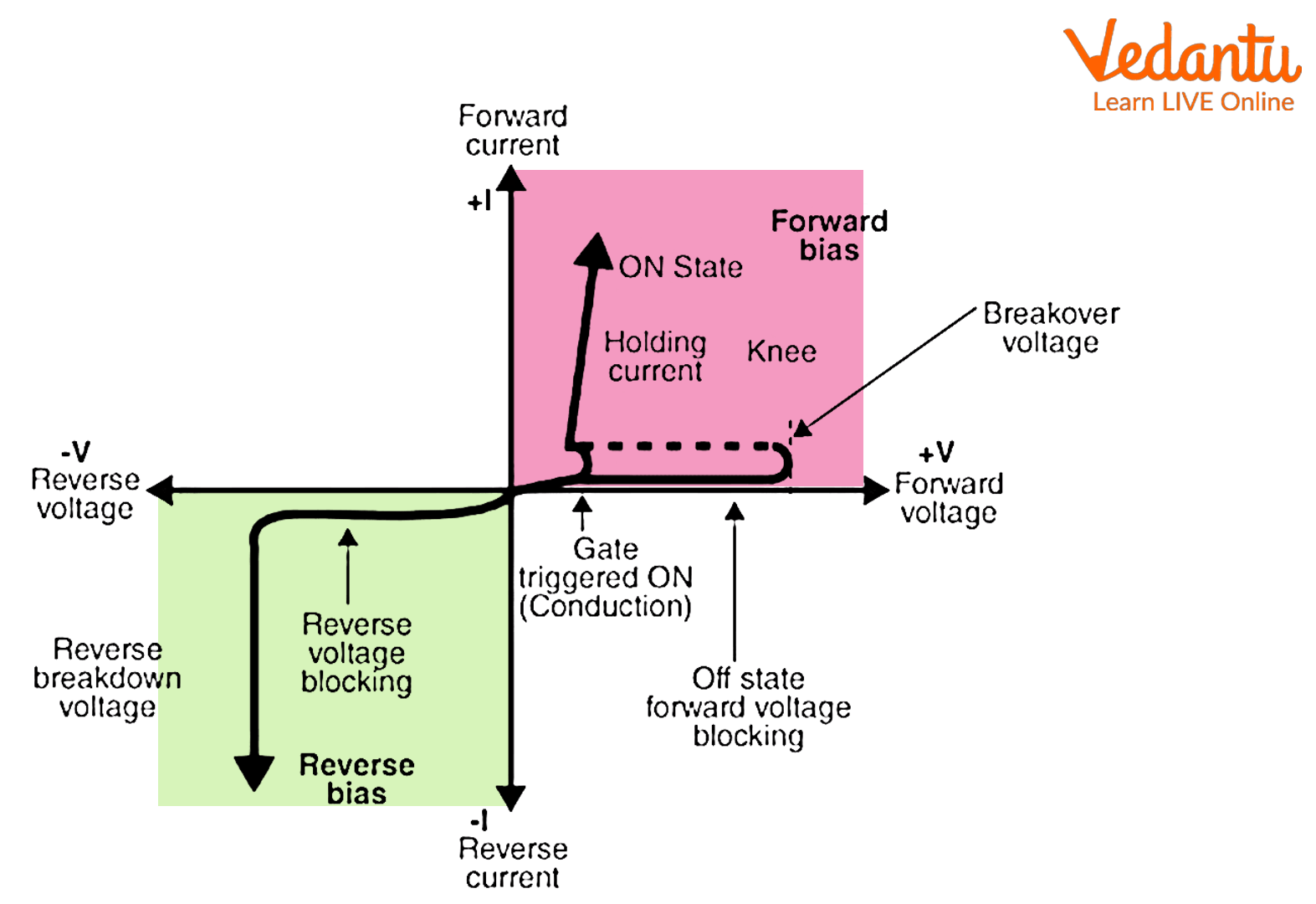V-I Characteristics of SCR

## V-I Characteristics of MOSFET

MOSFET is an abbreviation for Metal Oxide Semiconductor Field Effect Transistor.

It is a three-terminal device with a voltage output that regulates current flow between the output terminals, source, and drain. So they have drain (D), source (S), and gate (G) terminals, with a substrate (S) as the fourth terminal. The two types of power MOSFETs are depletion-type transistors and enhancement-type transistors.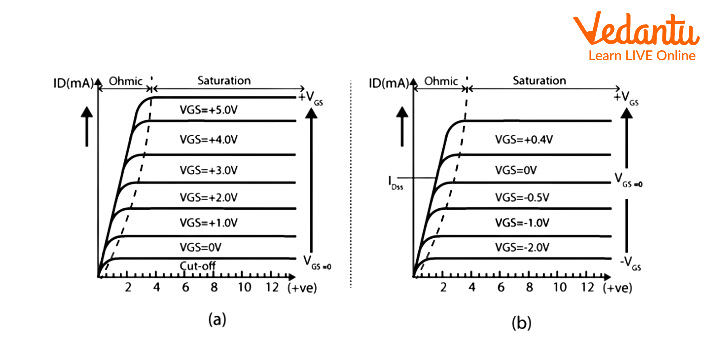V-I Characteristics of MOSFET

## V-I Characteristics of LED

LED is an abbreviation for light-emitting diode, which is used in all types of semiconductor diodes with electrical properties similar to a PN junction diode.

The LED V-I characteristic is as follows: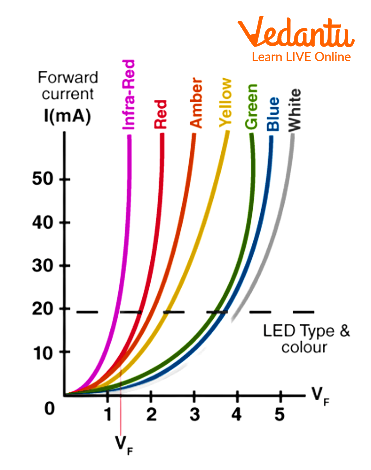V-I Characteristics of LED

## V-I Characteristics of PN Junction Diode

A PN junction diode is formed when an N-type material is fused with a P-type material to form a semiconductor diode. When an N-type material (which has more free electrons) is fused with a P-type material (which has more holes) to form a semiconductor diode. There are three types of biassing conditions:

• Zero bias

• Forward bias

• Reverse bias

The graph below depicts the p-n junction diode's V-I characteristics curve.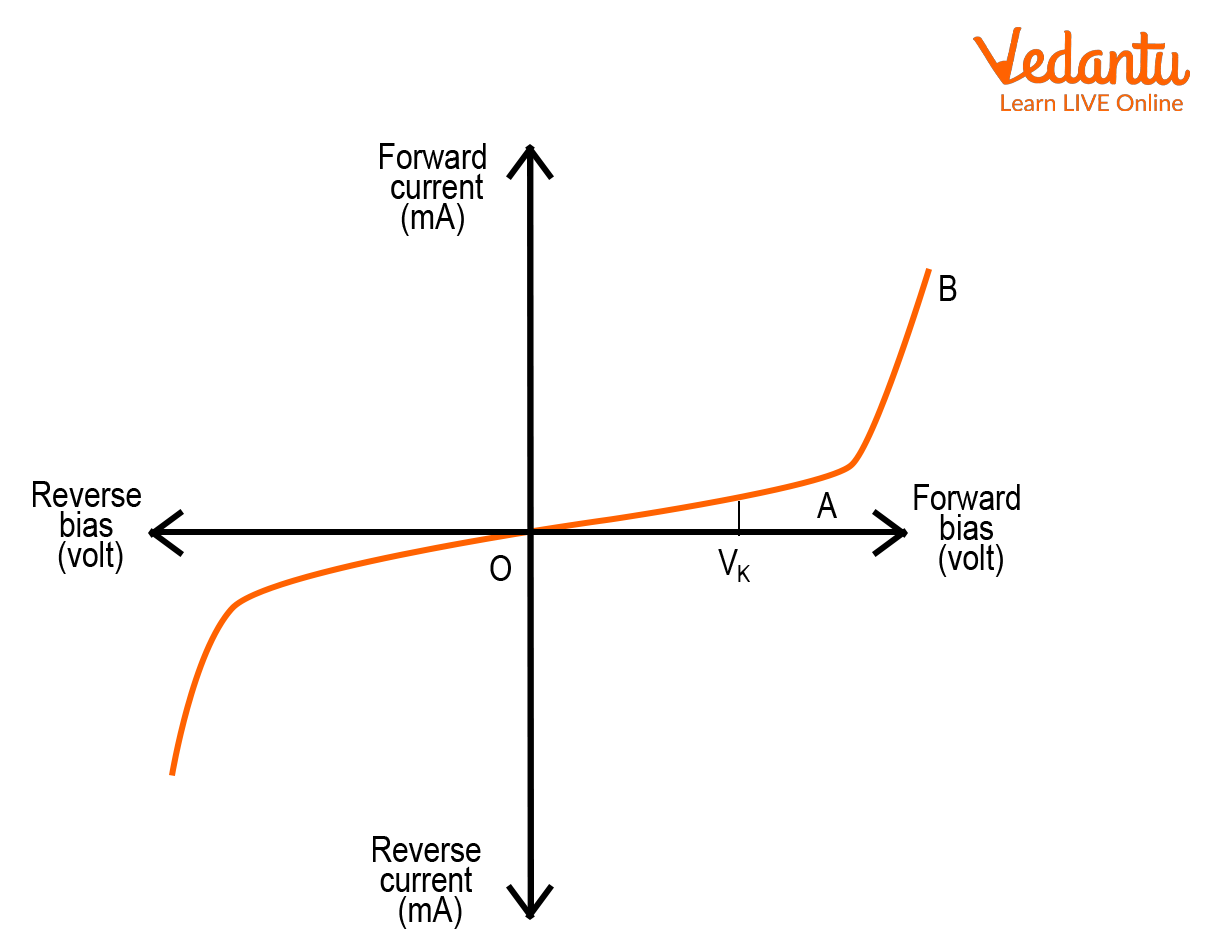V-I Characteristics of PN Junction Diode

## V-I Characteristics of Zener Diode

A Zener diode is an electronic device that operates in the Zener breakdown region. When these diodes are forward-biassed, they behave similarly to p-n junction diodes. Because they are heavily doped, these diodes have a very thin depletion region and can carry more electric current than regular p-n junction diodes.

The V-I characteristic of the Zener diode is given below: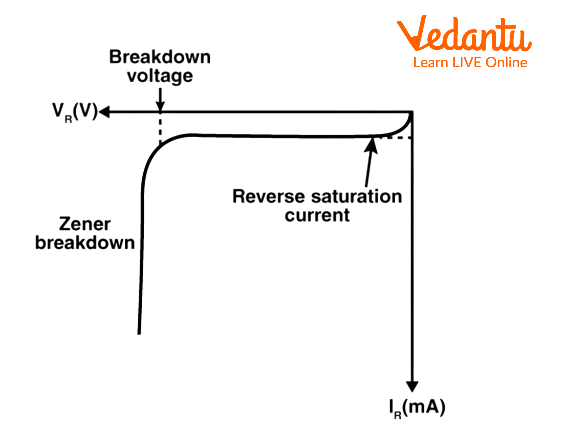V-I Characteristics of Zener Diode

## V-I Characteristics of Photodiode

The photodiode operates in reverse bias mode. Reverse voltages are plotted in volts along the X axis, and reverse currents are plotted in microamperes along the Y axis. Reverse current is independent of reverse voltage. When there is no light illumination, reverse current is almost non-existent. "Dark current" is the smallest amount of current present. When the brightness of the light increases, so does the reverse current.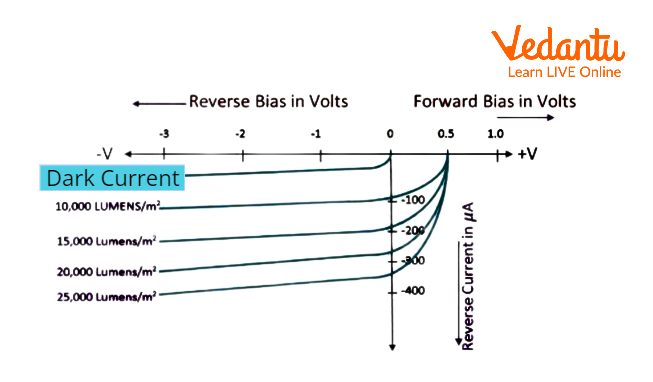V-I Characteristics of Photodiode

## V-I Characteristics of BJT

The VI characteristics of a power BJT differ from those of a signal-level transistor. The main distinctions are the quasi-saturation region and the secondary breakdown region. The device operation at the primary and secondary breakdown regions should be avoided because it will result in the device failing catastrophically.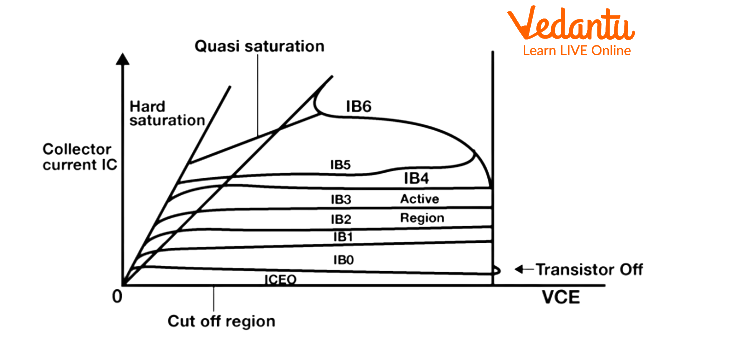V-I Characteristics of BJT

## Solved Problems

1. A transistor has a current gain of 30 Ampere. If the collector resistance is 6 kΩ, and the input resistance is 1 kΩ, calculate its voltage gain.

Solution:

Given,

${{R}_{in}}$ =1 kilo ohms and ${{R}_{out}}$ = 6 kilo ohms

${{R}_{gain}}$ = ${\dfrac{{R}_{out}}{{R}_{in}}}$ = ${\dfrac{{6}}{{1}}}$ = 6

Voltage gain = current gain $\times$ Resistance gain = 30 $\times$ 6 =180

2. A battery of 10 Volts connected to a conductor induces a current of 10 mA in the conductor. Find the resistance of the conductor.

Solution:

The relation gives the resistance of a conductor,

R = ${\dfrac{{V}}{{I}}}$

Given:

V = 10V

I = 10mA = 0.01 A

Plugging in the values inside the relation,

R = ${\dfrac{{V}}{{I}}}$

R = ${\dfrac{{10}}{{0.01}}}$

R= 1000 ohms

## Summary

V-I characteristics are the voltage or current characteristics of an electrical problem or device. The v-i graph provides useful information about the resistance and breaks down a digital component of the equation. It also serves as a workspace for an element. Reading an object's characteristics allows us to determine where and how to use it in an electric circuit. Electronic factors are distinguished by their voltage-ampere characteristics when applied to varying voltages. When a voltage is applied across an electronic component, it deviates from the graph that depicts the relationship between voltage and current when current is measured.

Last updated date: 28th May 2023
Total views: 239.7k
Views today: 4.37k

## FAQs on V-I Characteristics

1. What is meant by zero bias?

When no external voltage is provided to a PN junction diode, it achieves zero bias.

A zero-bias junction is also known as an unbiased PN junction. Because no external voltage is applied to the PN junction diode, electrons diffuse to the P-side while holes diffuse to the N-side and mix. As a result, these charge carriers generate an electric field. The electric field limits the further dispersion of charge carriers, resulting in no movement in the intermediate zone. This region is referred to as depletion width or space charge.

2. What are the VI voltage properties of a Zener diode?

As the reverse voltage supplied to the Zener diode approaches the desired Breakdown Voltage (Vz), a current begins to flow through the diode, known as the Zener Current, and the process is known as Avalanche Breakdown.

In response to an increase in reverse voltage, the junction in an enhancement-type transistor will break down at a specific amount of reverse voltage, resulting in a significant increase in reverse current flowing through the device. Zener breakdown is the name given to this type of breakdown.

3. What exactly is a diode, and the Construction of Diodes?

A diode is a two-terminal electronic component that primarily conducts current in one direction. It has a high resistance at one end and low resistance at the other, allowing the current to flow only one way. A diode is represented in an electrical circuit diagram by a triangle with a line running through one of its vertices.

Diodes can be manufactured of either silicon or germanium, the two semiconductor materials. The diode is considered forward-biased when the anode voltage is greater than the cathode voltage, and it conducts freely with a relatively low voltage drop. Similarly, when the cathode voltage is higher than the anode voltage, the diode is said to be reverse-biased.